﻿ 移相控制双向DC/DC变换器回流功率的优化

移相控制双向DC/DC变换器回流功率的优化Reflux Power Optimization of Phase-Shift Control Bidirectional DC/DC Converter

Abstract: The traditional bi-directional DC/DC converter with dual-phase shift control can produce large backflow power, especially when the voltage conversion ratio is mismatched, which will lead to high power loss and reduce system transmission efficiency. To solve this problem, this paper proposes an optimized phase-shifting control strategy to control the operation of the converter at the minimum reflux power point. In the case of different transmission power, the piecewise optimization algo-rithm is used to calculate the shift comparison combination under the minimum reflux power. The effectiveness of the control strategy and the accuracy of the theoretical analysis are verified by ex-periments.

1. 引言

2. 移相控制双向DC/DC变换器

2.1. 电路拓扑结构

$n={N}_{\text{1}}/{N}_{\text{2}}$ (1)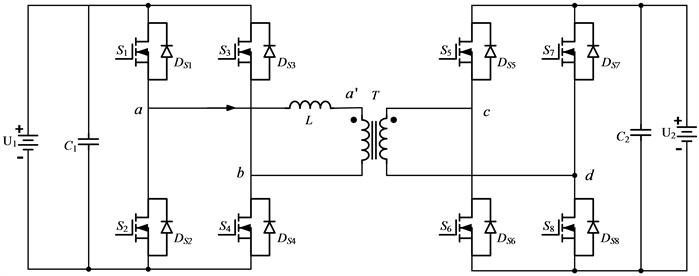Figure 1. Topology structure of bidirectional full-bridge DC/DC converter

2.2. 移相控制器的设计

DPS控制已经得到了大量的研究 ，其控制原理本文不再赘述。当内移相比D1外移相比D2存在 $\text{0}\le {D}_{1}\le {D}_{2}\le \text{1}$ 的关系，并且电压转换比 $k\ge 1$ 时，取SPS控制下的最大传输功率为基准值，DPS控制下变换器传输功率 ${P}_{D}$ 和回流功率 ${q}_{D}$ 的标幺值表达式为：

$\left\{\begin{array}{l}{P}_{D}^{*}=\text{2}{D}_{1}\left(2{D}_{2}-{D}_{1}-1\right)+\text{4}{D}_{2}\left(1-{D}_{2}\right)\\ {q}_{D}^{*}=\frac{{\left[-\left(k+2\right){D}_{1}+2{D}_{2}+k-1\right]}^{2}}{\text{2}\left(k+1\right)}\end{array}$ (2)

${D}_{2}=\frac{{D}_{1}+1-\sqrt{1-{P}_{D}^{*}-{D}_{1}^{2}}}{2}$ (3)

$\text{0}\le {D}_{1}\le \sqrt{1-{P}_{D}^{*}}$ (4)

${q}_{D}^{*}=\frac{{\left[-\left(k+\text{1}\right){D}_{1}+k-\sqrt{\text{1}-{P}_{D}^{*}-{D}_{1}^{2}}\right]}^{2}}{\text{2}\left(k+1\right)}$ (5)

$\frac{\text{d}{q}_{D}^{*}}{\text{d}{D}_{1}}=\frac{-\left(k+1\right){D}_{1}+k-\sqrt{1-{P}^{*}-{D}_{1}^{2}}}{k+1}\left(-k-1-\frac{-{D}_{1}}{\sqrt{1-{P}^{*}-{D}_{1}^{2}}}\right)$ (6)

$\left\{\begin{array}{l}{D}_{11}=\frac{{k}^{2}+k±\sqrt{2k+2-{P}_{D}^{*}\left({k}^{2}+2k+2\right)}}{{k}^{2}+2k+2}\\ {D}_{12}=±\frac{\left(k+1\right)\sqrt{\left(\text{1}-{P}_{D}^{*}\right)\left({k}^{2}+2k+2\right)}}{{k}^{2}+2k+2}\end{array}$ (7)

$\left\{\begin{array}{l}{P}_{D}^{*}\le \frac{2k+2}{{k}^{2}+2k+2}\\ {D}_{11}\le \sqrt{1-{P}_{D}^{*}}\\ {D}_{11}\le \frac{k}{k+1}\end{array}$ (8)Table 1. Internal shift ratio D1 at minimum reflux power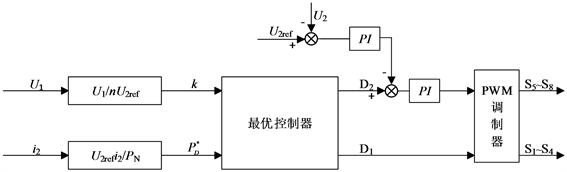Figure 2. Reflux power optimization block diagram

3. 实验验证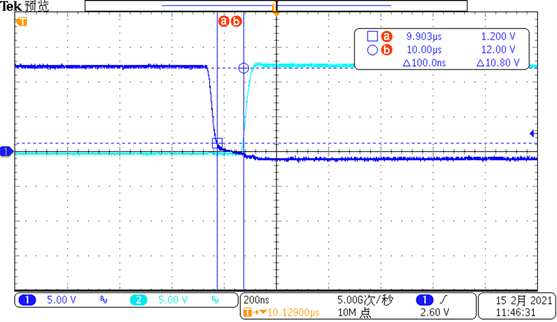Figure 3. Dead time of switching tube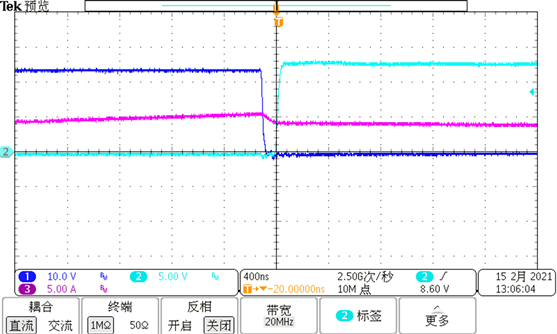Figure 4. MOS switching process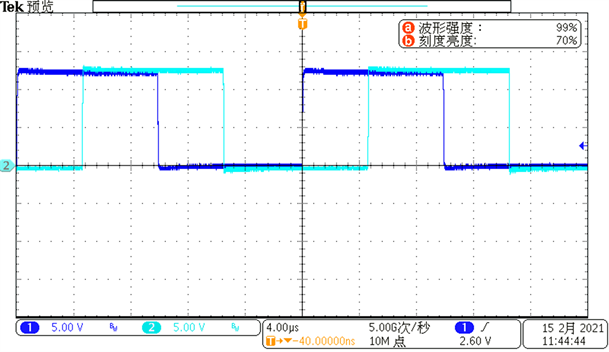Figure 5. The driving waveform of MOS tube S1 and S5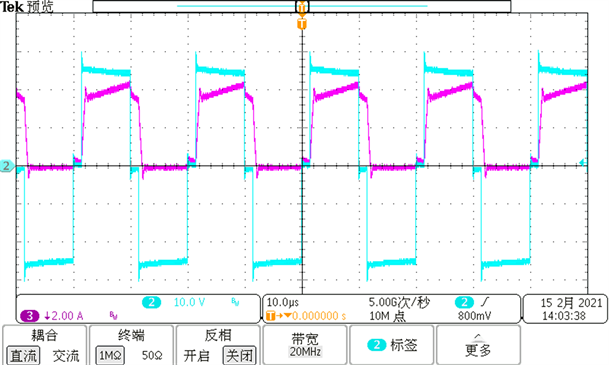Figure 6. Primary side output voltage and inductance current waveform

4. 结论

NOTES

*通讯作者。

 晏坤, 王辉, 漆文龙, 等. 微电网储能系统中基于PWM加双重移相控制的双向DC/DC变换器研究[J]. 电力自动化设备, 2015, 35(4): 44-52.

 Maciel, R.S., De Freitas, L.C., Coelho., E.A.A., et al. (2015) Front-End Converter with Integrated PFC and DC-DC Functions for a Fuel Cell UPS With DSP-Based Control. IEEE Transactions on Power Electronics, 30, 4175-4188.
https://doi.org/10.1109/TPEL.2014.2359891

 Mokrani, Z., Rekioua, D., Rekioua, T. (2014) Modeling, Control and Power Management of Hybrid Photovoltaic Fuel Cells with Battery Bank Supplying Electric Vehicle. International Journal of Hydrogen Energy, 39, 15178-15187.
https://doi.org/10.1016/j.ijhydene.2014.03.215

 赵彪, 于庆广, 孙伟欣. 双重移相控制的双向全桥DC-DC变换器及其功率回流特性分析[J]. 中国电机工程学报, 2012, 32(12): 43-50.

 刘赫, 路鑫, 高圣伟, 等. 双有源桥DC-DC变换器的回流功率优化控制[J]. 电源技术, 2020, 44(2): 277-280, 294.

 谷泓杰, 江道灼, 尹瑞, 等. 基于三重移相的双向全桥DC-DC功率特性分析[J]. 中国电力, 2016, 49(7): 122-127.

 孙凯, 陈欢, 吴红飞. 面向储能系统应用的隔离型双向DC-DC变换器分析方法与控制技术综述[J]. 电工电能新技术, 2019, 38(8): 1-9.

 Xu, G., Sha, D., Xu, Y., et al. (2018) Hybrid-Bridge-Based DAB Converter With Voltage Match Control for Wide Voltage Conversion Gain Application. IEEE Transactions on Power Electronics, 33, 1378-1388.
https://doi.org/10.1109/TPEL.2017.2678524

 郭华越, 张兴, 赵文广, 等. 扩展移相控制的双有源桥DC-DC变换器的优化控制策略[J]. 中国电机工程学报, 2019, 39(13): 3889-3899.

Top